# Kerala Syllabus 10th Standard Chemistry Solutions Chapter 3 Reactivity Series and Electrochemistry

You can Download Reactivity Series and Electrochemistry Questions and Answers, Summary, Activity, Notes, Kerala Syllabus 10th Standard Chemsitry Solutions Chapter 3 help you to revise complete Syllabus and score more marks in your examinations.

## Kerala State Syllabus 10th Standard Chemistry Solution Chapter 3 Reactivity Series and Electrochemistry

### Reactivity Series and Electrochemistry Text Book Questions and Answers

Reactivity Series And Electrochemistry Kerala Syllabus Text Book Page No: 48

→ Which metal reacts vigorously?
Sodium.

→ Which gas is formed as a result of this reaction?
Hydrogen.

→ Write down its chemical equation.
2Na + 2H2O → 2NaOH + H2

→ Complete the table (3.1) given below.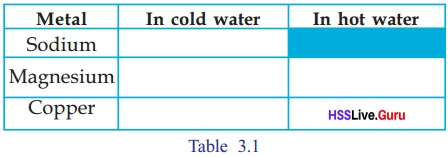→ Based on your observation, arrange these metals in the decreasing order of their reactivity.
Sodium > Magnesium > Copper
→ 2Mg + O2 →
2Mg + O2 → 2MgO

Sslc Chemistry Chapter 3 Kerala Syllabus Text Book Page No: 49

→ Which metal among magnesium, copper, gold, sodium and aluminium, loses its lustre at a faster rate?
Sodium

→ List the above metals in the decreasing order of their reactivity with air and thereby losing lustre
Sodium > Magnesium > Aluminium > Copper > Gold.

Text Book Page No: 50

→ What happened to the Zn rod?
Before the experiment the Zn rod was colourless. After the experiment Zn rod became blue due to the deposition of copper.

→ What is the reason for this?
When the Zn rod is dipped in CuSO4 solution, the Cu2+ ions in the solution get deposited at the Zn rod as Cu atoms.

→ What is the reason for the change in intensity of the colour of CuSO4 solution?
The blue colour of CuSO4 solution is due to the presence of Cu2+ ions. The change in intensity of the colour of CuSO4 solution because when the Zn rod is dipped in CuSO4 solution, the Cu2+ ions in the solution get, deposited at the Zn rod as Cu atoms.

→ Which is the metal that gets displaced here?
Copper

→ Which is more reactive Zn or Cu?
Zn

→ On the basis of the position of Zn and Cu in the reactivity series, can you explain why Cu had been displaced?
Zn is placed above Cu in the reactivity series because Zn has a higher reactivity than Cu.

→ Isn’t it due to the higher reactivity of zinc (Zn) when compared to copper (Cu)?
Yes.

Chemistry Class 10 Chapter 3 Kerala Syllabus Text Book Page No: 51

→ Is this reaction oxidation or reduction? Why?
Oxidation. Because the losing of electrons is called oxidation.

→ The change that happened to Cu2+
Cu–2+ + 2e → Cu

→ What is the name of this reaction? Why?
Reduction. The gaining of electrons is called reduction.Complete this chemical equation by assigning oxidation numbers.
2 Ag+1 NO31–+ Cu°→ Cu2+ (NO3)–12 + 2Ag0

→ Which metal was oxidised in this case? Which metal was reduced?
Metal which was oxidised: Cu
Metal ion which was reduced: Ag+

→ Write equations showing oxidation and reduction.
Oxidation : Cu0 → Cu2+ + 2e
Reduction : Ag++ le → Ag0

Reactivity Series And Electrochemistry Sslc NotesText Book Page No: 52

→ Complete the table 3.3.Sslc Chemistry Chapter 3 Notes Pdf Kerala Syllabus Text Book Page No: 53

→ Which electrode has the ability to donate electrons in a cell constructed using these metals?
Zn

→ Which one can gain electrons?
Cu

→ Identify the chemical reaction that takes place at the Zn electrode. Tick ✓ the right one.
Zn(s) → Zn2+ (aq) + 2e  (✓)
Zn2+(aq) + 2e → Zn(s)   (✘)

→ What is the reaction taking place here?
Oxidation.

→ Write the chemical equation for the reaction taking place at the Cu electrode.
Cu2+ (aq) + 2e → Cu(s)

→ Sketch the cell constructed.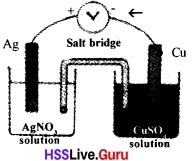→ Note down the reaction of the Galvanic cell.
Cu(s) + 2Ag+(aq) → Cu2+ (aq) + 2Ag(s)
Cu(s) → Cu2+(aq) + 2e (Anode)
Ag+(aq) + le → Ag(s) (Cathode)

→ Direction of flow of electrons From Cu to Ag
Mark the direction of electron how in the cell illustrated.

→ write the reactions taking place at cathode and anode.
At cathode : Ag+ + le → Ag
At anode : Cu → Cu2+ + 2e

Hss Live Guru 10th Chemistry Kerala Syllabus Text Book Page No: 55

→ You have used three metals Zn, Cu and Ag. How many cells can be produced using these?
Three.

→ Complete the Table 3.4 by writing anode and cathode in each.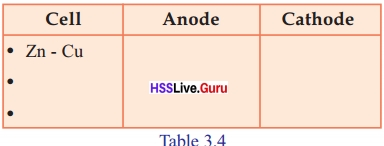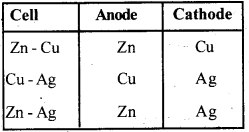→ What are the substances obtained when electricity is passed through acidified water?
Hydrogen, Oxygen.

→ Do such type of chemical changes happen when electricity is passed through metals?
Yes.

Sslc Chemistry Chapter 3 Questions And Answers Kerala Syllabus Text Book Page No: 56

→ To which electrodes are the positive ions attracted during electrolysis?
Towards negative electrodes(Cathode)

→ To which electrodes are the negative ions attracted?
Towards positive electrodes(Anode),

→ What changes happen to the ions which N are attracted to cathode?
Reduction

→ What about the changes happening to the ions attracted to anode?
Oxidation

→ Which ion is attracted to the positive electrode (anode)?
Chloride ion (Cl)

→ What is the chemical reaction taking place there?
2Cl (aq) → Cl2(g) + 2e

Text Book Page No: 57

→ Which is the gas liberated at the anode?
Chlorine(Cl2)

→ Which is the ion attracted to the negative electrode (cathode)? Write the change happening to it?
Na+ ions. These ions accept one electron and changes to sodium atom. That is sodium ions are reduced.

→ Which is the metal deposited at the cathode?
Sodium (Na)

→ Which are the ions attracted to the positive electrode?
Ans.
Cl,OH

→ Which are the ions attracted to the negative electrode?
Na+,
H3O+,
H2O.

Text Book Page No: 59

→ Which metal is connected to the negative terminal of the battery?
Iron.

→ Which metal is connected to the positive terminal of the battery?
Copper.

→ Which solution is used as the electrolyte?
Copper sulphate solution.

→ What happens to Cu2+ ions at the cathode? Complete the equation.
Cu2+ + 2e → Cu

→ What happened to the copper ions? Oxidation/Reduction?
Reduction.

→ Complete the equation given below.
Cu → Cu2+ + 2e

Text Book Page No: 60

→ Find out more examples and extend the list.

• Chromium plating is used in motor car etc.
• To make metal coating easily corroding metals to prevent corrosion.
• In ICs (Integrated Circuits) coating of gold /silver is made by electroplating.

### Reactivity Series and Electrochemistry Let Us Assess

Kerala Genetics Question 1.
The solutions of ZnSO4, FeSO4, CuSO4 and AgNO3 are taken in four different test tubes. Suppose, an iron nail is kept immersed in each one
In which test tube the iron nail undergoes a colour change?
What is the reaction taking place here?
Iron nail immersed in solution of CuSO4 and AgNO3 undergoes a colour change.
i. Fe(s) + CuSO4(aq) →
FeSO4 (aq) + Cu (s).
ii. Fe(s) + 2AgNO3 (aq) →
Fe(NO3)2(aq) + 2Ag(s).
Iron displaces Cu from CuSO4 and Ag from AgNO3 because Fe has higher reactivity than Cu and Ag.

Genetic Engineering Syllabus Question 2.
Compare the electrolysis of molten potassium chloride and solution of potassium chloride. What are the processes taking place at the cathode and the anode?
Molten KCl
KCl (s) → K+ + Cl
At the negative electrode:
K+ + le → K (reduction – cathode)
At the positive electrode:
2Cl → Cl2 + 2e (oxidation- anode)
Solution of potasium chloride.
At the negative electrode:
2H2O + 2e → H2 + 2OH (cathode).
At the positive electrode:
2Cl → Cl2 + 2e (anode).

Future Diary 10th Question 3.
You are given a solution of AgNO3, a solution of MgSO4, a Ag rod and a Mg ribbon. How can you arrange a Galvanic cell using these? Write down the reactions taking place at the cathode and the anode.
At anode,
Mg (s) → Mg2+ (aq) + 2e
At cathode,
Ag (aq) + le → Ag (s)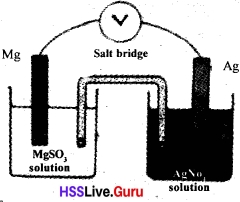### Reactivity Series and Electrochemistry Extended Activities

The Reactivity Question 1.
1. Keep two carbon rods immersed in copper sulphate solution. Then pass electricity through the solution.
i. At which electrode does colour change occur anode or cathode?
ii. Is there any change in the blue colour of the copper sulphate solution?
iii. Write down chemical equations for the changes occurring here.
i. At cathode
iii.At cathode: Cu2+ (aq) + 2e → Cu (s)
At anode: 2H2O → O2(g) + 4H+ (aq) + 2e

SSLC Chemistry Chapter 4 Question 2.
When acidified copper sulphate solution is electrolysed oxygen is obtained at the anode. What arrangements are to be made for this? Find the element deposited at the cathode.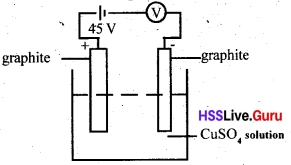Element deposited at the cathode: Copper.

Question 3.
a. When Galvanic cells are made using the metals like Mg, Cu, Zn and Ag, what will be the nature of reactions in each cell?
(Reactivity: Mg > Zn > Cu >Ag)
b. How many Galvanic cells can be made by using the metals Ag, Cu, Zn and Mg?
a. i. Cu-Ag cell
Anode: Cu (s) → Cu2+ (aq) + 2e
Cathode: Ag+ (aq) + le → Ag(s)
ii.Zn-Ag cell
Anode: Zn (s) → Zn2+ (aq) + 2e
Cathode: Ag+ (aq) + le → Ag (s)
(iii) Mg-Ag cell
Anode: Mg (S) → Mg2+ (aq) + 2e
Cathode: Ag+ (aq) + le → Ag (s)
(iv) Zn – Cu cell
Anode: Zn (s) → Zn2+ (aq) + 2e
Cathode: Cu2+ (aq)+ 2e → Cu(s)
(v) Mg-Cu cell
Anode: Mg (s) → Mg2+ (aq) + 2e
Cathode: Cu2+ (aq) + 2e → Cu (s)
(vi) Mg-Zn cell
Anode: Mg (s) → Mg2+ (aq) + 2e
Cathode: Zn2+(aq) + 2e → Zn(s)
b. 6 cells

### Reactivity Series and Electrochemistry Orukkam Questions and Answers

Scope of Genetic Engineering Question 1.
1. Take cold water and Hot water in two test tubes, Add one or two drops of phenolphtha lein in it. Drop equally sized Mg ribbon in it.
a. In which test tube pink colour occured sharply?
b.Why did pink colour appear in that test tube so early?
c. Which gas evolved out from both test tubes?
d. Write balanced equation for the above mentioned reaction.
a. Pink colour occurred sharply in the test tube with hot water.
b. Temperature is a factor that affects the rate of a reaction. When heated the kinetic energy of molecules increases and hence the rate of chemical reaction also increases which causes the pink colour to appear early.
c. Hydrogen
d. Mg(s) + 2H2O(l) → Mg(OH)2(aq) + H2(g)

SCERT Question Pool 2017 Question 2.
Cut a small sodium metal piece into two, watch it.
a. What change occurred on the surface of sodium metal?
b.Write one word for the process of this type of decomposition.
c. Write down the equations for this.
a. After some time the cut piece of sodium will turn dull.
b.Corrosion.
c. 4Na(s) + O2(g) → 2Na2O
2Na(s) + 2H2O(l) → 2NaOH(s) + H2(g)
2NaOH (s) + CO2(g) → Na2CO3(s) + H2O(l)

Question 3.
Take equal quantities of dil HCl in five test tubes. Drop Mg, Zn, Fe, Cu in each test tube. Watch carefully.
a. Arrange metals in decreasing order of reactivity.
b.Write balanced equations for each reaction.
a. Mg > Zn > Fe > Cu
b. Zn + 2HCl → ZnCl2 + H2
Fe + 2HCl → FeCl2 + H2
Cu + HCl → No reaction
Mg + 2HCl → Mg Cl2 + H2

SCERT Question Pool Question 4.
Some metals and metallic compounds are given in the table. If the metal substitute the metal in the compound put a tick mark in the corresponding column and otherwise a cross mark in the column. Write down correct answer based on the table given below.

 Metalsolution Mg Cu Zn Ag Fe CuSO4 × × ZnSO4 × × × × AgNO4 × MgSO4 ×

a. Correct the table if necessary.
b. Is it possible to substitute lower positioned metals by top positioned metals in the reactivity series?
c. What type of reaction is this?
d. Write down balanced equations for all the true sign given in the table.
a.

 Metalsolution Mg Cu Zn Ag Fe CuSO4 ✓ × ✓ × ✓ ZnSO4 ✓ × × × × AgNO4 ✓ ✓ ✓ × ✓ MgSO4 ✓ ✓ ✓ × ✓

b.Yes. It is possible.
c. Substitution reactions.
d.CuSO4 + Mg → Cu + MgSO4
CuSO4 + Zn → ZnSO4+ Cu
CuSO4 + Fe → FeSO4 + Cu
ZnSO4 + Mg → MgSO4 + Zn
AgNO3 + Mg → Ag + MgNO3
AgNO3 + Cu → CuNO3 + Ag
AgNO3 + Zn → ZnNO3 + Ag
AgNO3 + Fe → FeNO3 + Ag

Human Insulin Gene Question 5.
Draw maximum number of Galvanic cell using substances given in the table.
Salt bridge, Zinc rod, Copper rod, Voltmeter, Aluminium chloride, Copper sulphate, Zinc sulphate, Silver nitrate, Silver rod, Calcium chloride
a. Complete the table based on the figures drawn.

 Galvanic Cell Electrode which Gives Electron Electrode which Gain Electron

B. Write down the general names used for an electrode which gives electrons,
c. Metals in that electrode in the reactivity series is (in the Top, Bottom)
d. General name of the Electrode which accepts electron.
e. Process of giving electron is
f. Process of Accepting electron is
g.Direction of the flow of Electron
h.Write down the balanced equation taking place in both electrodes.

 Galvanic Cell Electrode which Gives Electron Electrode which accepts Electron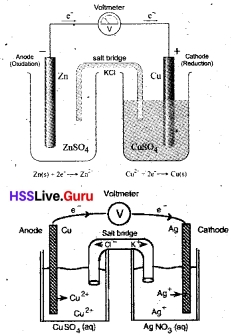Zn-Ag cell is also possible.
a.b. Anode.
c. In the top.
d. Cathode.
e. Oxidation.
f. Reduction.
g. From Anode to Cathode.Chemistry Reactivity Series Question 6.
Take Cupric chloride (CuCl2) solution in a beaker. Dip two graphic rod in it. Pass 5V electricity through it.
a. Why electricity passes through cupric chloride solution?
b.Which gas evolved out through positive electrode? How did you identify that gas?
c. Which product formed in negative electrode?
d. In which electrode oxidation and reduction take place?
e. Write one word for the process of chemical change happening in a Electrolyte while passing Electricity?
a. Electrolytes are substances which conduct electricity in molten states or in aqueous solutions. In molten state ions of CuCl2 can more freely. These ions are responsible for the Conduction of electricity by electrolytes.
b.Chlorine.
c. Copper.
d.Oxidation takes place at the positive electrode and Reduction takes place at the negative electrode.
e. Electrolysis.

Reactivity Series Class 10 Question 7.
Take 25 ml water in a beaker and the pass electricity through it. Then add little sulphuric acid in it.
a. Why electricity didn’t pass through pure water?
b. What happens when dil H2SO4 is added?
c. Which type of ion formed more when sulphuric acid is added in water?
d. Complete the equation of the Ionization 0f H2SO4
H2SO4 → 2H+ +
Based on the equation given below write down the correct answers.
2H+ +  + 2H2O → 2H3O +  + SO42–
e. Complete the equation.
f. Write down the name of H3O+ ion?
g.Which ion is moving towards negative ion?
h.Complete the reaction taking place in the negative electrode.
2H3O+(aq) + 2e →  +
i. Whicfrion is having highest oxidation potential?
j. Complete the reaction taking place in positive electrode.
2H2O →  + 4H+
k. Ions remain in the beaker after the electrolysis are
I. What product form when these two combined together
a. Since the number of ions is less, pure water does not allow the passage of electricity.
b. When dil H2SO4 is added the water becomes a good electrolyte. Hence electricity passes through it. When a little dilute sulphuric acid is mixed with water large quantity of hydronium ions are formed.
c. Hydronium ion (H3O+)
d. H2SO4 → 2H+ + SO42–
e. 2H+ + S042– + 2H2O → 2H3O+ + SO42–
f. Hydronium ion
g. H3O+
h. 2H3O+(aq) + 2e → H2(g) + 2H2O
i. H2O has the highest oxidation potential when compared to SO42–
j. 2H2O → O2(g) + 4H+ + 4e
k. 4H+, SO42–ions.
l. H2SO4 is formed when these 2 combine together.

Reactivity Series Chemistry Question 8.
a. Complete the table based on the Electrolysis of molten sodium chloride.

 Electrodes Reaction taking place product Anode Cathode

b. Write down the reaction taking place in each electrodes and products formed in the electrolysis of sodium chloride solution.

 Electrodes Reaction taking place product Anode Cathode

c. Why is hydrogen formed in the cathode instead of sodium?
d. Write one word for a solution undergoes chemical change when electricity passes through it.
e. Write the name of the above process.
f. Write down the uses of above type of reaction.c. When Na+ ion and water are compared reduction occurs to water. Hence H2 is liberated at cathode.
H++ e → H,
H + H → H2.
d. Electrolyte.
e. Electrolysis.
f. Electroplating, Production of chemicals, Purification of metals.

### Reactivity Series and Electrochemistry Evaluation Questions

Take little water in a test tube add two drops of phenolphthalein in it Same quantity of Kerosene is added to the mixture and a small piece of sodium is dipped in it.

Insulin Gene Question 1.
What kind of colour formed in the test tube? Why?
Water become pink in colour. Because when phenolphthalein is added to water it becomes alkaline.

Question 2.
Which gas is bubbled on the surface of sodium metal?
Hydrogen

Question 3.
Write balanced equation of the reaction between sodium and water.
2Na(s) + 2H2O(l) → 2NaOH (aq) + H2(g).

Question 4.
What products occurs when Iron reacts with water vapour?
Fe3O4 (Iron Oxide) and Hydrogen gas.

Question 5.
Lustre of magnesium disappeared fast when it placed in open space why?
When Magnesium is kept in open space it reacts with atmosphere air and a light coat of magnesium oxide is formed. This is the reason for Magnesium to lose its lustre.

Question 6.
Verdigris formed on copper utensils disappeared after some days why?
The copper in copper utensils react with atmospheric air and forms copper oxide. Due to this verdigris are formed on Copper utensils.

Question 7.
Lustre of aluminium utensils disappear after some days. Why?
Aluminium reacts with atmospheric air and Aluminium oxide is formed. This process takes place slowly. Hence Aluminium utensils loose their lustre.

Question 8.
Write down the equation for the reaction between CuSO4 and iron nail? What type of reaction is this?
CuSO4 + Fe → FeSO4 + Cu.
Redox reaction.

### Reactivity Series and Electrochemistry SCERT Questions and Answers

Question 1.
5ml water is taken in 3 test tubes. Copper, sodium and magnesium of equal mass are dropped in different test tubes. Test tubes having copper and magnesium are heated.
a. Write the observations in the heated test tubes.
b. Write the equation for the reaction in the test tube in which sodium is dropped,
c. Arrange these metals in the decreasing order of their reactivity.
a. Mg reacts with hot water liberating hydrogen, Copper does not react with hot water.
b. 2Na + 2H2O → 2NaOH + H2.
c. Na > Mg > Cu.

Question 2.
a. Which metal among copper, aluminium and gold loose its metallic lustre at a faster rate? Write the equation of the reaction.
b. Sodium is kept in Kerosene. Why?
a. Aluminium, Al + 3O2 → 2Al2O3
b. Na reacts with air (oxygen) and water.

Question 3.
An experimental setup is made to compare the reactions of Mg, Zn and Cu with dilute hydrochloric acid.
a. Write the procedure and observation of the reaction.
b. Which is the gas evolved when zinc reacts with dilute hydrochloric acid?
a. Take Mg, Zn and Cu in different test tubes and add dilute HCl to each.
Observation: Magnesium and Zinc reacts with dilute hydrochloric acid copper does not react with the acid.
b.Hydrogen.

Question 4.a. What are the changes that can be observed with the iron rod and the colour of copper sulphate solution?
b. Write the equations of the oxidation and reduction reactions.
c. What will be the change if silver rod is used instead of iron rod? What is the reason?
a. Copper is deposited on iron and the blue.colour of copper sulphate solution decreases.
b. Cathode – Cu2+(aq) + 2e → Cu (s) (Reduction)
Anode – Fe (s) → Fe2+(aq) + 2e (Oxidation)
c. No change occurs, Reactivity of silver is less than that of copper. In the reactivity series, the position of silver is below copper.

Question 5.
Sodium reacts with water.
a. Identify the gas evolved in the reaction h If two drops of phenol[ihthaloin is added to the water, what will be colour change of the resultant solution? Explain the reason?
a. Hydrogen.
b. Colour changes to pink. Due to the presence of sodium hydroxide (alkaline nature).

Question 6.
Three Galvanic cells are given.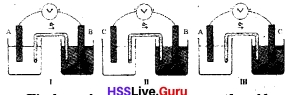a. Find out the most reactive metal and least reactive metal among them.
b. In cell, which electrode undergoes oxidation why?
c. Write the equation of the redox reaction occurring in cell 3 (Valency of A, B are 2.)
a. Most reactive metal A, Least reactive metal B.
b. A Reactivity of A is higher than B.
c. A+ 2e → A2+,
C2+ + 2e → C ,
A + C2+ → A2+ C .

Question 7.
Some metals and salt solutions are given (Cu, Zn, Ag, ZnSO4, AgNO3, MgCl2)
a. Draw the diagram of a Galvanic cell that can be made using these substances.
b. Find out the anode and cathode of this cell and write the chemical equation for the reaction at cathode.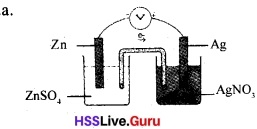b. Anode – Zn,
Cathode – Ag
2Ag++ 2e→ 2Ag

Question 8.
Give reasons for the following.
a. Iron vessels are not used a boilers that are used to boil water.
b. Blue vitriol solution is not kept in iron vessels.
a. Iron reacted with steam-heated to high temperature

Question 9.
Examine the given electrolytic cell.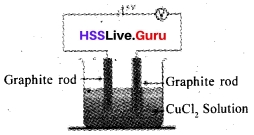a. Which gas is evolved at the positive electrode?
b. Write the oxidation and reduction reactions of this cell.
c. What is the difference in the energy transformation of a Galvanic cell and an electrolytic cell?
a. Chlorine / Cl2
b. 2Cl → Cl2 + 2e
Cu2+ + 2e → Cu
c. Galvanic cell – Chemical energy is converted to electrical energy.
Electrical Cell – Electrical energy is changed to chemical energy.

Question 10.
The solutions in the given table electrolyzed.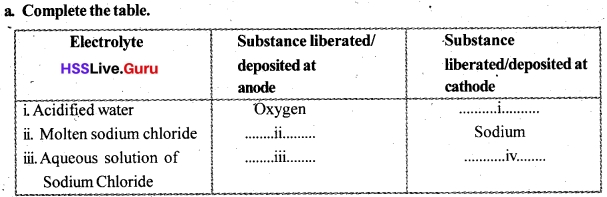List any two areas in which electrolysis is made use of?
a. i. Hydrogen.
ii. Chlorine.
iii.Chlorine.
iv. Hydrogen.
b.

• Purification of metals.
• Electroplating.
• Production of chemicals.

Question 11.
The position of iron is below that of zinc in reactivity series. The cell formed by them is given. Correct the mistakes and redraw.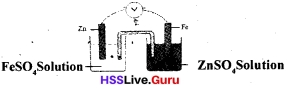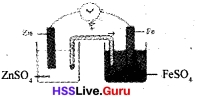Question 12.
Sodium chloride solution is electrolysed using platinum electrodes.
a. Write the chemical equation of the reaction at cathode.
b. What happens when phenolphthalein is added to the solution? State the reason?
a. 2H2O + 2e → H2 + 2OH
b. Colour turns pink. Presence of sodium hydroxide in the solution.

Question 13.
The anode and cathode of two Galvanic cells are given.

 Galvanic Cell Anode Cathode Cell 1 Mg Zn Cell 1 Zn Ag

A. Mg → Mg2+ + 2e
B. Zn2++2e → Zn
C. Ag+ +le → Ag
D. Zn → Zn2+ + 2e
E. Ag → Ag+ + le
F. Mg2+ +2e → Mg
a. Find out the reactions at the anode and cathode for each cell from the above.
b. Which metal can act only as cathode? Why?
a. Cell 1: Anode Mg → Mg2+ + 2e
Cathode Zn2+ + 2e → Zn
Cell 2: Anode Zn → Zn2+ + 2e
Cathode Ag+ + le → Ag
b. Ag. Lesser tendency to give up electron that is it is a less reactive metal.

Question 14.
The chemical reactions of various Galvanic cells are given as incomplete in the table. Complete them.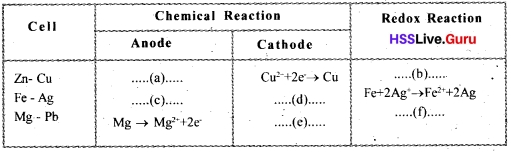a. Zn → Zn2++ 2e
b. Zn+Cu2+ → Zn2+ + Cu
c. Fe → Fe2+ + 2e
d. 2Ag+ + 2e- → 2Ag
e. Pb2++ 2e → Pb
f. Mg + Pb2+ → Mg2+ + Pb

Question 15.
The picture of a Galvanic Cell is given below.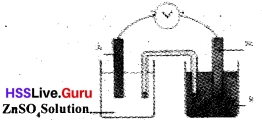a. Identify A and B.
b. Give the direction of electron flow?
c. Write the chemical equation at the anode and cathode.
a. A – Zn,
B – FeSO4Solution
b. From Zn to Fe
c. Anode Zn → Zn2+ + 2e
Cathode Fe2+ + 2e → Fe

Question 16.
An incomplete table about the electrolysis of different electrolytes are given below. Complete it.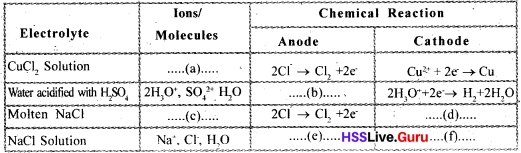a. Cu2+,
Cl,
H2O.
b. 2H2O → O2 + 4H+ + 4e
c. Na+,
Cl
d. Na+ + le → Na
e. 2Cl → Cl2 + 2e
f. 2H2O + 2e→ H2 + 2OH

Question 17.
5mI AgNO3 is taken in a test tube and a copper rod is dipped into
a. Identify the changes occurring with the copper rod and the solution?
b. Complete the equation of the reaction.
Cu + 2AgNO3 →  +
c. Write the equations of the oxidation and reduction reactions.
a. Silver is deposited in copper rod. Colour of solution changes to blue.
b. Cu + 2 AgNO3 → Cu(NO3)2 + 2Ag
c. 2Ag+ + 2e → 2Ag (Reduction)
Cu → Cu2+ + 2e (Oxidation)

### Reactivity Series and Electrochemistry Exam Oriented Questions and Answers

Very Short Answer Type Questions (Score 1)

Question 1.
The symbols of certain metals are given below. Arrange them as they are given in the reactivity series.
Mg, Pb, Ag, Cu, Zn, Fe, Au, Sn.
Mg, Zn, Fe, Sn, Pb, Cu, Ag, Au.

Question 2.
Analyse the table given below and answer the questions.a. Find out the metals which are likely to be A, B and C from the box given below.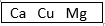b. Write down the chemical equation between metal ‘B’ and water.
a. A – Mg,
B – Cu,
C – Ca
b. Cu (s) + 2H2O (l) → Cu OH2 (aq) + H2 (g)

Very Short Answer Type Questions (Score 2)

Question 3.
Certain metals are given below:
Ag, Zn, Pb, Sn, Fe
a. When a galvanic cell is constructed using these metals, which one acts only as anode? Give the reason.
b. Draw Zn-Fe cell. Mark the direction of electron flow and write the chemical equation anode.
a. Zn. Because Zn has a higher reactivity than the other 4 metals.Direction of electron flow from Zn to Fe Chemical reaction at anode:
Zn(s) → Zn2+(aq) + 2e

Question 4.
Zn (s) + 2AgNO3 (aq) →
Zn(NO3)2 (aq) + 2Ag
a. Write the oxidation state of each element in this displacement reaction.
b. Write the chemical equation for oxidation and reduction.
a. Zn° (s) + 2Ag1+N5+ O32– (aq)
→ Zn2+(N5+O2–3)2 (aq) + 2Ag°

b.Oxidation:
Zn° (s) → Zn2+ (aq) + 2e
(Oxidation means losing of electrons)

Reduction:
Ag1+ (aq) + le → Ag (s)
(Reduction means gaining of electrons)

Question 5.
Based on the reactions given below, answer the following questions.
i. Aqueous solution of CuCl2 undergoes electrolysis using graphite rods.
ii. Molten KCl undergoes electrolysis.
iii. Aqueous solution of NaCI undergoes electrolysis.
a. In which all reactions Cl2 gas is formed? At which electrode is Cl2 gas formed?
b. In which reaction H2 gas is formed? Write the chemical equation of this reaction.
a. Cl2 gas is formed in all the three reactions. Chlorine gas is formed at anode.

b. When aqueous solution of NaCI undergoes electrolysis, hydrogen gas is formed at cathode.
Equation: 2H2O + 2e → H2 + 2OH

Very Short Answer Type Questions (Score 3)

Question 6.
Take water in 4 different beakers and add a small piece of sodium, lead, iron and copper in each.
a. In which all solutions gas bubbles will be formed? Which gas is formed?
b. Which solution will turn pink on adding phenolphthalein? Why?
c. Write the chemical equation between this metal and water.
a. Gas bubbles are formed in the beaker containing sodium. Hydrogen is tlie gas formed.

b. Beaker containing sodium turns pink because sodium reacts with water and forms an alkali, sodium hydroxide. Alkalies turn phenolphthalein pink.

c. 2Na (s) + 2H2O (l) → 2NaOH (aq) + H2 (g)

Question 7.
Analyse the picture given below.a. Identify ‘A’.
b. Write the chemical equation at ‘B’.
c. Add few drops of phenolphthalein to the remaining solution after electrolysis. What change will take place? Why?
a. A – cathode.

b. Chemical equation at ‘B’:
2Cl → Cl2 + 2e

c. Solution turns pink. Because, after electrolysis K+ and OH ions are present in the remaining solution. That means KOH, an alkali is formed.

Question 8.
The flow of electron in certain galvanic cells are given below:
i. Cu → Ag
ii. Ag → Zn
iii.Na → Mg
iv.Fe → K
a. Choose the incorrect ones.
a. ii. Ag → Zn,
iv. Fe → K are the incorrect ones.

b. Zn has a higher reactivity than Ag. Hence Zn undergoes oxidation (loses electrons). So the direction of electron flow is from Zn to Ag. Similarly, K has higher reactivity than Fe. Hence direction of electron flow is from K → Fe.

Question 9.
Which of the chemical reactions given below are wrong? Explain the reason.
a. Cu (s) + 2HCl (aq) →
CuCl2(aq) + H2 (g)
b. Mg(s) + Pb(NO3)2 (aq) →
Mg(N03)2 (aq) + Mg (s)
c. 3Fe (s) + 4H2O (l) →
Fe3O4(s) + 4H2(g)
Equations (a) and (c) are wrong.
a. Copper cannot displace hydrogen from acids because it is placed below hydrogen in the reactivity series.

c. Fe reacts only with superheated steam. Fe does not react with water in liquid state.

Question 10.
Write the chemical equation of the electrolysis of water to which little sulphuric acid (H2SO4) is added.
H2SO4 (l) + 2H2O (l) → 2H3O (aq) + SO22–(aq)
at cathode:
2H3O+ (aq) + 2e → H2(g) + 2H2O (l) (reduction)
at anode:
2H2O (l) → O2(g) + 4H (aq) + 4e (oxidation)

Long Answer Type Questions (Score 4)

Question 11.
Analyse the reactions and answer the following questions: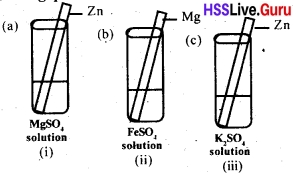a. Which among the following test tubes will undergo a chemical reaction?
b. What are these chemical reactions called?
c. Explain the oxidation and reduction reactions taking place here including the chemical equation?
a. Chemical reaction takes place in test tube (ii) only.
b. These chemical reactions are called displacement reactions. .
c. Chemical equation: (including oxidation states)
Mg° (s) + Fe2+S6+O42– (aq) →
Mg2+S6+O2–4 (aq) + Fe° (s)
At Mg : Mg°(s) → Mg2+(aq) + 2e (oxidation)
At Fe2+, Fe2+ (aq) + 2e →  Fe°(s) (reductipn)

Question 12.
A students observation is given below:
i. When Zn is put in salt solution, Na gets deposited over Zn.
ii. Au reacts with water vapour and hydrogen gas is formed.
iii. Al reacts with acid and forms hydrogen gas.
iv. Mg reacts with hot water and forms hydrogen gas.
a. Which statements are incorrect?
a. Statements (i) and (ii) are wrong.

b. i. Zn cannot displace sodium. Because sodium has a higher reactivity than Zn.
ii. Au does not react with water vapour.

Question 13.
“Sodium cannot be kept open in atmospheric air, and cannot be stored in water. So it is stored in kerosene.” Give explanation for the above statement with its chemical equation.
Sodium which is placed above in the reactivity series reacts vigorously with water and oxygen.
4Na (s) + O2 (g) → 2Na2O (s)
2Na (s) + 2H2O (l) →
2NaOH (aq) + H2(g)
This NaOH reacts with CO2 in the atmospheric air and forms sodium carbonate.
2NaOH (s) + CO2 (g) → Na2CO3 + H2O (l)
To avoid the reaction with O2, H2O and CO2, sodium is stored in kerosene.

Question 14.
The direction of electron flow in certain galvanic cells are given below. (Symbols are not real)
i. B → A,
ii. E → C,
iii.D → E,
iv.A → D.
a. Arrange the metals A, B, C, D and E in the decreasing order of their reactivity.
b. Choose the reaction taking place at ‘C’ in cell (ii) E → C. Give the reason.
i. C+ (aq) + le → C(s)
ii. C (s) → C+ (aq) + le
iii.C (s) → C+ (aq) + le
a. B,
A,
D,
E,
C.
b.C+ (aq) + le → C (s) is the correct equation. Because ‘E’ has higher reactivity than. So ‘C’undergoes reduction.

Question 15.
Give reason for the following.
a. CuSO4 solution is not stored in iron vessels.
b. Buttermilk is not stored in aluminium vessels.
Ans.
a. Fe displaces copper from copper sulphate solution and forms FeSO4.
b. Aluminium reacts with acid in the buttermilk.